# Circumcenter

Circumcenter

There is a neighborhood in Seattle, called the Denny Triangle, because of its triangular shape.

Imagine that you and your two friends live at each vertex of the Denny Triangle. You plan a meeting this weekend at a point that is equidistant from each of your homes.

Do you know that by using perpendicular bisectors to find the circumcenter of this triangle, you can exactly locate your meeting point? Isn't that interesting?

Let's learn more about this!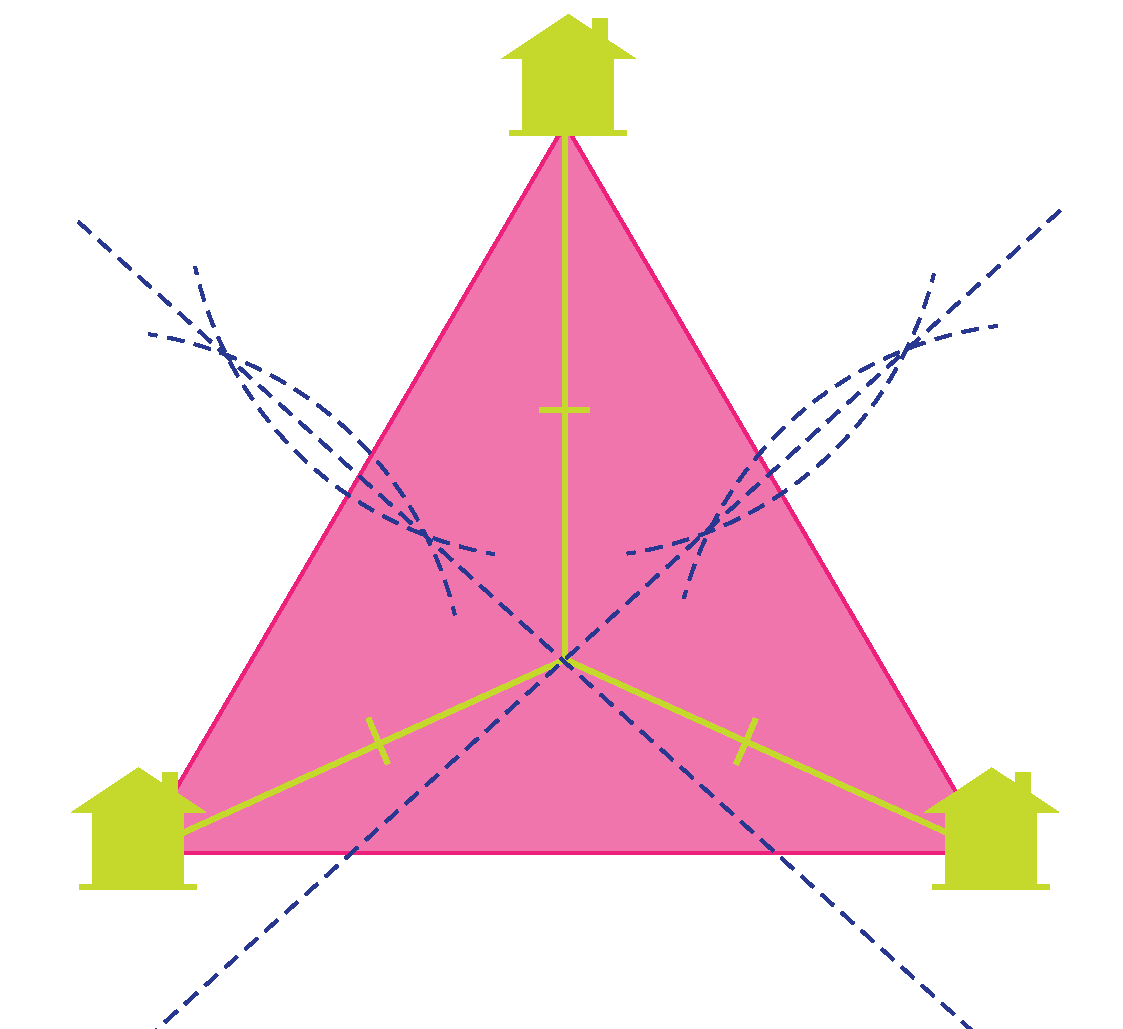In this mini-lesson, we will learn all about circumcenter. The journey will take us through properties, interesting facts, and interactive questions on circumcenter.

## Lesson Plan

 1 What Is a Circumcenter? 2 Important Notes on Circumcenter of a Triangle 3 Thinking out of the box! 4 Solved Examples on Circumcenter 5 Interactive Questions on Circumcenter

## What Is a Circumcenter?

### Circumcenter Definition

The circumcenter is the center point of the circumcircle drawn around a polygon. The circumcircle of a polygon is the circle that passes through all of its vertices and the center of that circle is called the circumcenter. All polygons that have circumcircle are known as cyclic polygons.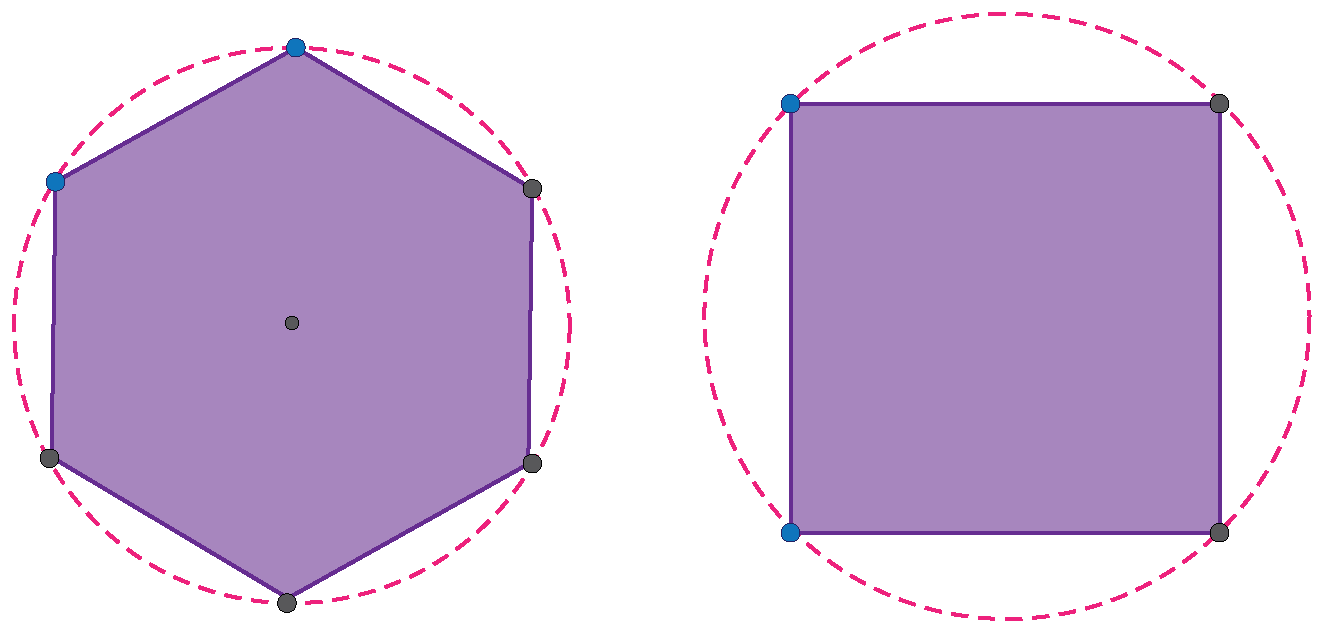However, all polygons need not have the circumcircle. Only regular polygons, triangles, rectangles, and right-kites can have the circumcircle and thus the circumcenter.

### The Circumcenter of a Triangle

All triangles are cyclic and hence, can circumscribe a circle, therefore, every triangle has a circumcenter. The circumcenter of a triangle can be found out as the intersection of the perpendicular bisectors (i.e., the lines that are at right angles to the midpoint of each side) of all sides of the triangle. This means that the perpendicular bisectors of the triangle are concurrent (i.e. meeting at one point).

## Construction of a Circumcenter

### Circumcenter Geometry

Steps to construct the circumcenter of a triangle:

Step 1: Draw the perpendicular bisectors of all the sides of the triangle using a compass.

Step 2: Extend all the perpendicular bisectors to meet at a point. Mark the intersection point as $$\text O$$, this is the circumcenter.

Step 3: Using a compass and keeping $$\text O$$ as the center and any vertex of the triangle as a point on the circumference, draw a circle, this circle is our circumcircle whose center is $$\text O$$.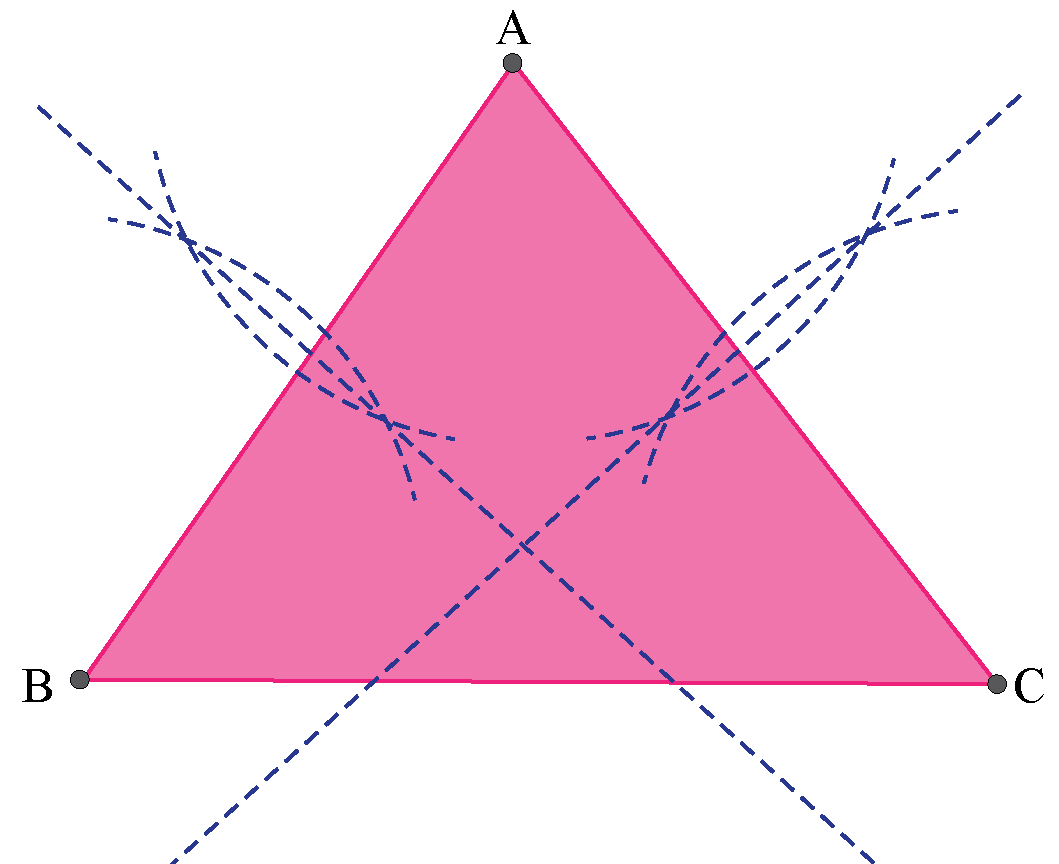You can construct a circumcenter using the following simulation.

Just place the D end of the compass at the center of the hypotenuse of the triangle and end E at one of the vertex.

Press Draw circle and circumcenter will be drawn by the simulator.

## Properties of a Circumcenter

Consider any$$\triangle \text {ABC}$$ with circumcenter $$\text O$$.

1. All the vertices of the triangle are equidistant from the circumcenter.

Join $$\text O$$ to the vertices of the triangle.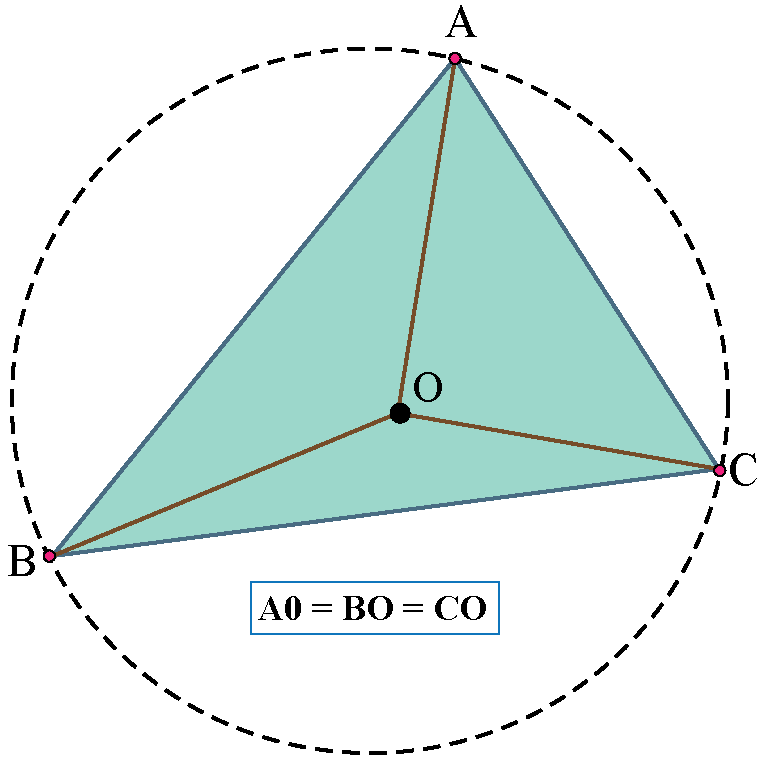$AO = BO = CO$(radius of the same circle)

Hence, the vertices of the triangle are equidistant from the circumcenter.

2. All the new triangles formed by joining $$\text O$$ to the vertices are Isosceles triangles.

3. Angle $$\angle \text {BOC} = 2\angle \text A$$ when $$\angle \text A$$ is acute or when $$\text O$$ and $$\text A$$ are on the same side of $$\text {BC}$$.

4. Angle $$\angle \text {BOC} = 2( 180^{\circ} - \angle \text A)$$ when $$\angle \text A$$ is obtuse or $$\text O$$ and $$\text A$$ are on different sides of $$\text {BC}$$.

5.  Location for the circumcenter is different for different types of triangles.

$$Triangle$$  $$Location$$
Acute Angle Triangle Inside the triangle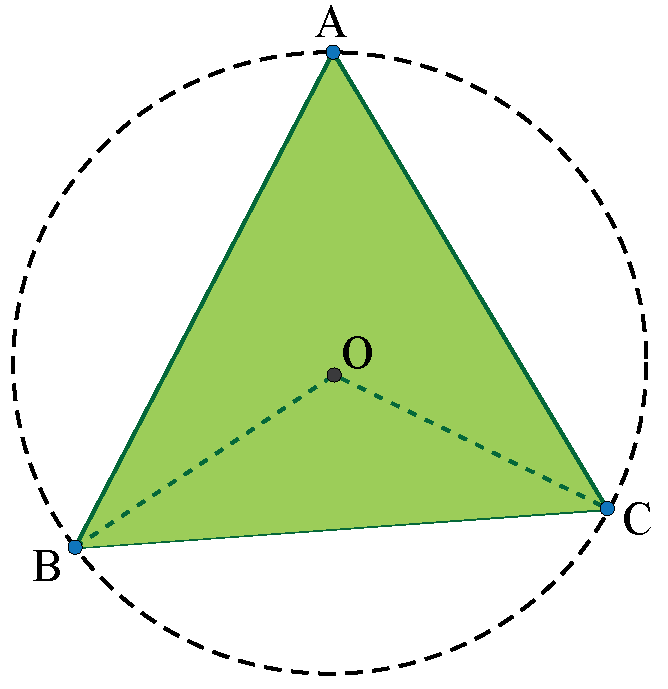Obtuse Angle Triangle Outside the triangle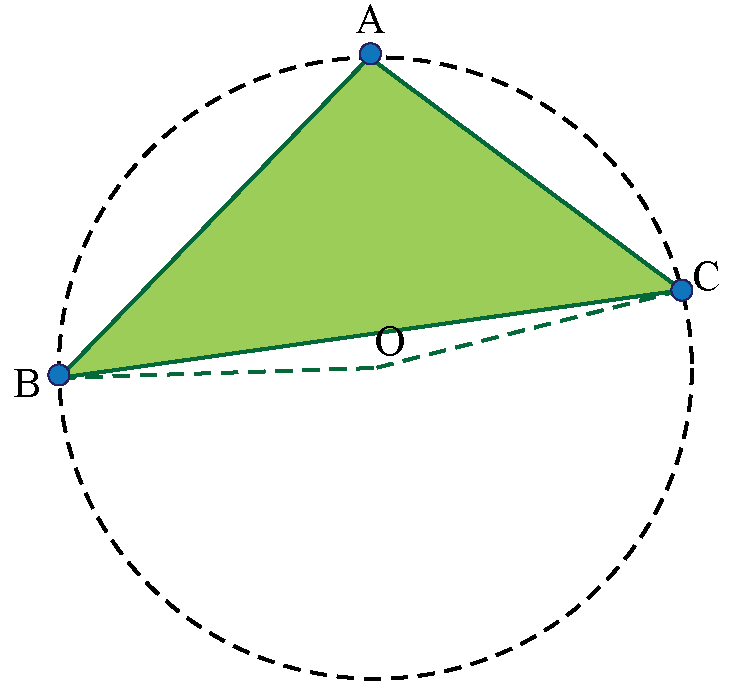Right Angled Triangle On the Hypotenuse of the triangle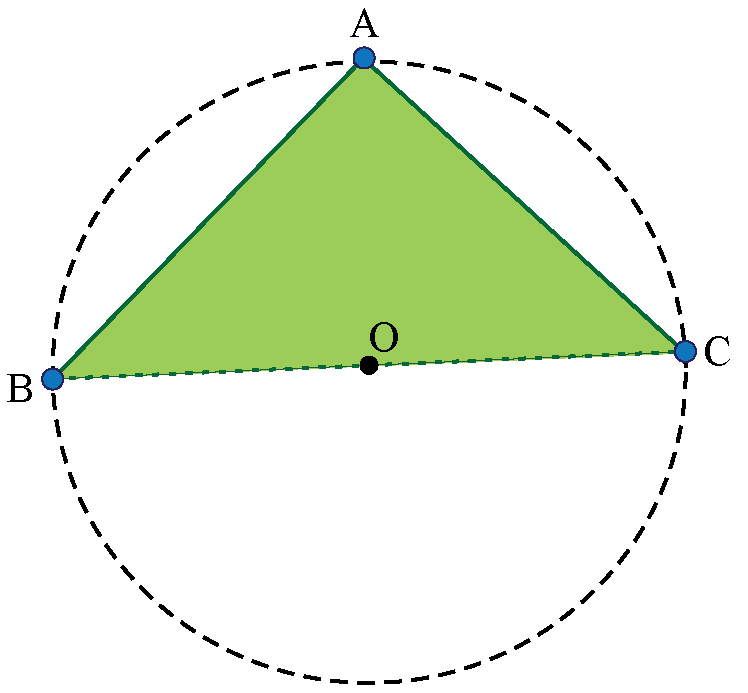More Important Topics
Numbers
Algebra
Geometry
Measurement
Money
Data
Trigonometry
Calculus